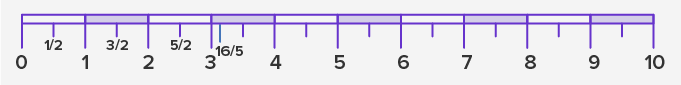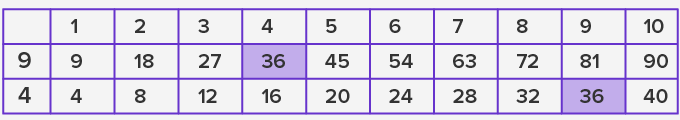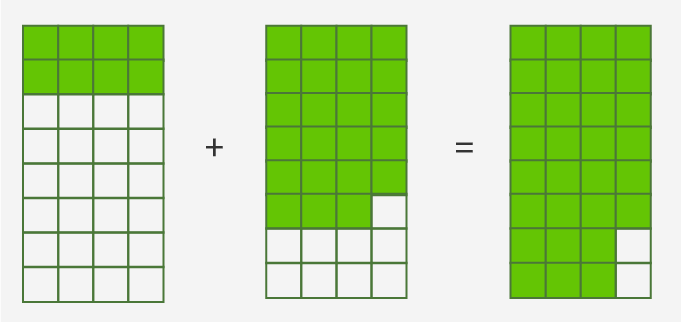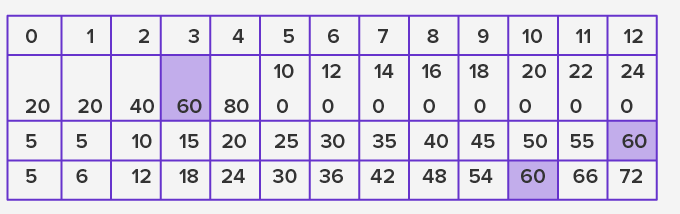# Least Common Denominator - Definition with Examples

The Complete K-5 Math Learning Program Built for Your Child

• 40 Million Kids

Loved by kids and parent worldwide

• 50,000 Schools

Trusted by teachers across schools

• Comprehensive Curriculum

Aligned to Common Core

## Least Common Denominator

### Fractions

Fractions are the numbers between two integers written in the form ofpq. We express these numbers in the form of a quotient or fraction of two integers.In a fraction, the “p” is the numerator and “q” is the denominator. The value of “q” must be a non-zero integer. For a unit fraction, “p” is always equal to “1”.

To understand it better, let’s find the numbers on the number line as follows:The number 0.8 lies between 0 and 1 and the number 2.5 lies between 2 and 3. We can also write them as:

0.8 = 8 10 , which can be simplified as  4 5

2.5 =  25 10  , which can be simplified as  10 2

The number  5 2  is an example of improper fraction where the numerator > denominatorFractions 1 2 , 3 2 , 5 2 and 16 5 marked on a number line.

## Least common denominator

The least common denominator is the smallest number of all the common multiples of the denominators when 2 or more fractions are given. Let’s add two fractions.

= 29 + 34

Since adding them will be difficult as the denominators are not the same, thus we need to find a common number to simplify it. For this, list the multiples of the number 9 and 4 in a table. The first common smallest multiple will be the least common denominator for the given fractions.Here, the least common multiple for 9 and 4 is 36. Thus, the expression can be written as:

=   2 9 + 3 4 = 2 9 x 4 4 + 3 4 x 9 9

= 836 + 2736

=  29 x 44 + 34 x 99

=  3536## Ordering fractions (least to greatest)

Using the least common denominator, fractions can be arranged in ascending or descending order.

For example, to arrange the following numbers in ascending order, we find their LCD.

3 5 , 9 20 , 4 6Using the table of multiples above, the LCD will be 60. Thus numbers can be rewritten as:

36 60 , 27 60 , 40 60

27 60 < 36 60 < 40 60

## Application

The concept of least common denominator for fractions is useful to evaluate the result as a part of the whole. In creating a solution using chemicals, the measurements using a cup, tube or flask, etc. are marked with fractions to maintain precision. Several areas in chemical science, physics, currency exchange, computing interest, time duration, etc. use fractions to represent the values.

 Fun Facts During 1800 BC, Egyptians used hieroglyphs (picture-based writing) of a mouth (represents a part) to represent unit fractions Romans used words to represent fractions with base 12, such as “Uncia” for 1 12 , “Semis” for 6 12 , etc.

## Related math vocabulary

• Multiples and multiplication

• Least Common Multiple

• Number operations

• Fractions

• Number ordering

Won Numerous Awards & Honors